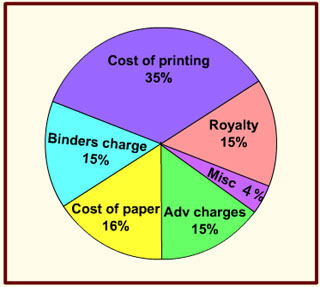### Examination Questions in Data Interpretation - 1

###### Click on the option you think is right and then check by clicking on the Show Answer button. You can change your option as many times as you like.

The following graph shows the expenditure incurred in bringing a book, by a magazine producer. Study the graph and answer the questions given below.1. What should be the central angle of the sector for the cost of the paper ?

1. 57.6°
2. 54.4°
3. 56.7°
4. 54.8°

2. If the miscellaneous charges are Rs. 6,000, the cost of paper is

1. Rs. 12,000
2. Rs. 18,000
3. Rs. 15,000
4. Rs. 24,000

3. If 5500 copies are published, miscellaneous expenditures amount to Rs. 1,848, find the cost price of 1 copy.

1. Rs. 10.40
2. Rs. 9.40
3. Rs. 12.40
4. Rs. 8.40

4. The cost of printing 100 copies was Rs. 10000/-, but the miscellaneous expenses just got doubled. What percentage of the total expenses are miscellaneous expenses?

1. 6.58%
2. 7.24%
3. 7.69%
4. 8.51%